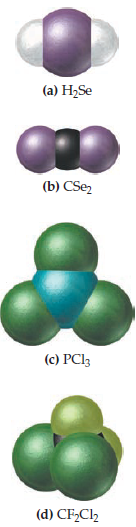Introductory Chemistry - 5 Edition - Chapter 10 - Problem 115p
Register Now

Join StudySoup

Get Full Access to Introductory Chemistry - 5 Edition - Chapter 10 - Problem 115p9780321910295

# Solved: Examine the formulas and space-filling models of the molecules shown here

Introductory Chemistry | 5th Edition

Problem 115P

Examine the formulas and space-filling models of the molecules shown here. Determine whether the structure is correct. If the structure is incorrect, sketch the correct structure.Accepted Solution
Step-by-Step Solution:
Step 1 of 3

Structure of H2Se and CS2:Structure of PCl3:Structure of CF2Cl2:###### Chapter 10, Problem 115P is Solved

Step 2 of 3

Step 3 of 3

Unlock Textbook Solution